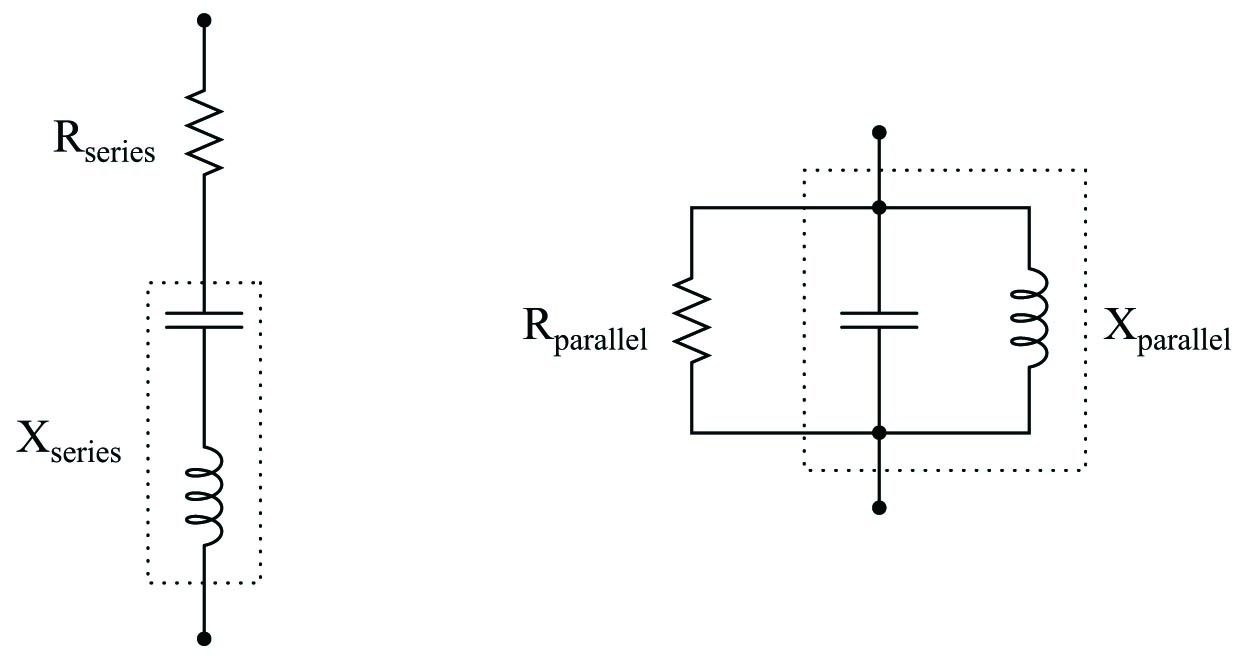# Equivalent Series and Parallel Circuits

## Chapter 5 - Basic Alternating Current (AC) Theory

Impedance in a series circuit is the orthogonal sum of resistance and reactance:

$Z = \sqrt{R^2 + (X_L - X_C)^2}$

Equivalent series and parallel circuits are circuits that have the exact same total impedance as one another, one with series-connected resistance and reactance, and the other with parallel-connected resistance and reactance. The resistance and reactance values of equivalent series and parallel circuits may be expressed in terms of those circuits’ total impedance:If the total impedance of one circuit (either series or parallel) is known, the component values of the equivalent circuit may be found by algebraically manipulating these equations and solving for the desired $$R$$ and $$X$$ values:

$Z^2 = R_{series}R_{parallel} \hbox{\hskip 100pt} Z^2 = X_{series}X_{parallel}$

Published under the terms and conditions of the Creative Commons Attribution 4.0 International Public License